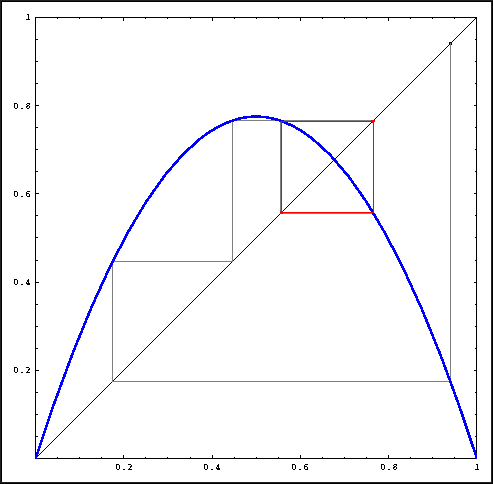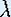Up: Iteration

# One-Dimensional Dynamical Systems

## Part 3: Iteration

#### Graphical iteration

Another, generally more preferred, way of visualizing orbits is based on the graph of the function that represents the dynamical system. It starts by showing the graph of a function y = f(x) and the graph of the identity function y = x. Suppose we have an orbit:

x0, f(x0), f(f(x0)), ... = x0, x1, x2, ...

We can visualize this orbit in the (x, y)-plane by drawing lines between points on the graph of f and points on the line y = x. The following sequence represents the same orbit as above:

(x0, x0), (x0, x1), (x1, x1), (x1, x2), (x2, x2), ...

Points of the form (xn, xn) are on the graph of the identity function, that is, on the line y = x. The points (xn, xn+1) = (xn, f(xn)) are on the graph of f.Visualizing an orbit with graphical iteration for the Logistic map.

Starting with (x0, x0) on the diagonal, draw a vertical line to the graph of f. Recall that f(x0) is the next point in the orbit of x0. Next, draw a horizontal line from (x0, f(x0)) to the diagonal. This way, we have repositioned our point so that a vertical line from this new position will give us f(f(x0)). This procedure, called graphical iteration, makes visualization easier and provides a geometric shortcut; you can even sketch an orbit instead of computing it.

Graphical iteration on the computer can be done with the following software. If you are using a Macintosh computer, follow the instructions for the software Chaos and Dynamics. On the other machines, you should follow the instructions for using Mathematica.

• Use the Java Applet Iteration of the Logistic Map to see how the dynamics close to the (positive) fixed points depends on the parameter. For each, which point is attracting? Are there the same number of attracting points for every?

• For this question you can either use Devaney's Chaos Lab #2, Mathematica or the Java Applet The Period Doubling Route to Chaos. In the latter case, follow the abstracted instructions.

Fix the starting point x0 = 0.1. For several choices ofbetween 0 and 3.5, investigate the eventual behavior of x0 = 0.1. In Devaney's Chaos Lab #2 you can push the Transient button to see what the eventual behavior is; in the other cases, the eventual behavior is shown in red.

Draw a diagram withon the horizontal axis and x on the vertical axis. For eachvalue that you checked, place a dot at the x values that are part of the eventual behavior of x0 = 0.1. If you checked the eventual behavior for enoughvalues, you should be able to sketch the curves connecting the dots, showing the continuous dependence on. Do not go beyond= 3.5.

Up: IterationThe Geometry Center Home Page

Written by Hinke Osinga